## Assorted forces, and applying Newton's laws

9-22-99

Sections 4.6 - 4.7

Forces can come from various sources. Whenever two objects are touching, they usually exert forces on each other, Newton's third law reminding us that the forces are equal and opposite. Two objects do not have to be in contact to exert forces on each other, however. The force of gravity, for instance, is a good example of a force that can arise without two objects being in contact with each other.

### Weight

Mass and weight are often used interchangeably, but they are quite different. The mass of an object is an intrinsic property of an object. If your mass is 50 kg, you have a mass of 50 kg no matter where you go: on Earth, on the Moon, in orbit, wherever. Your weight, on the other hand, will vary depending on where you are. Your weight is the magnitude of the gravitational force you experience, and it has units of force, Newtons. Because the gravitational force you experience on the Earth is different from that you'd experience on the Moon or in orbit, your weight would be different even though your mass remains constant.

We'll examine the force of gravity in more detail later in the course. For now, it's sufficient to remember that, at the surface of the Earth, the gravitational force on an object of mass m has a magnitude of mg. The direction is toward the center of the Earth.

### The normal force

Many forces do come from objects being in contact with each other. A book rests on a table: the book exerts a downward force on the table, and the table exerts an equal-and-opposite force up on the book. We call this force the normal force, which doesn't mean that other forces are abnormal - "normal" is the technical physics word for perpendicular. We call it the normal force because the force is perpendicular to the interface where the book meets the table.

The normal force is just one component of the contact force between objects, the other component being the frictional force. The normal force is usually symbolized by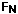. In many cases the normal force is simply equal to the weight of an object, but that's because in many cases the normal force is the only thing counter-acting the weight...that is not always the case, however, and one should always be careful to calculateby applying Newton's second law.

### The tension force

Whenever we use a rope (or something equivalent, like a string) to exert a force on an object, we're creating tension in the rope that transmits the force we exert on the rope to the object at the other end of the rope. The tension force is usually labeled by T. To makes our lives simpler, we usually assume that the rope has no mass, and does not stretch. Using these assumptions, when we exert a certain T on our massless unstretchable rope, the rope exerts that same T on the object. The rope itself will feel like it's being pulled apart, because we'll be exerting a certain T in one direction and the object we're pulling on will be exerting an equal-and-opposite force at the other end.

One rule to remember - you can't push with a rope. The tension force always goes along the rope away from the object attached to it.

### Applying Newton's laws

A good way to see how Newton's laws are applied is to do an example.

Example - A box of mass 3.60 kg rests on an inclined plane which is at an angle of 15.0° with respect to the horizontal. A string is tied to the box, and it exerts a force of 5.00 N up the ramp. What is the acceleration? Give the magnitude and direction.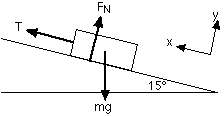The first step in solving this is to draw a picture, showing the box, inclined plane, and all the forces. Then choose a coordinate system, showing positive directions. One axis of your coordinate system should be aligned with the acceleration, which in this case is either up or down the ramp. Here, we've assumed that the box accelerates up the ramp, although we're not sure. This could be worked out by figuring out the component of the weight down the slope and comparing it to the tension force, but it's fine to guess. If we guess wrong we'll get a minus sign for the acceleration at the end which will tell us that the box accelerates down the slope...the magnitude will be correct, though.

The next step is to draw the free body diagram, which is similar to the first picture but without the inclined plane. Another difference is that the force of gravity has been split up into components parallel to the incline and perpendicular to the incline. In other words, its been split into its x and y components.Applying Newton's second law in the y-direction gives: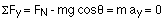The box is not accelerating in the y-direction, which is why the net force in that direction is zero. Solving for the normal force gives: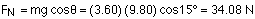Applying Newton's second law in the x-direction gives: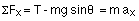Solving for the acceleration gives:

ax = T/m - g sin15° = 5.0/3.60 - 9.80 sin15° = -1.15 m/s2

So, the magnitude of the acceleration is 1.15 m/s2. The minus sign means that the box accelerates down the slope, rather than up as we'd assumed. This is because the component of the weight down the slope is larger than the tension.

### The problem-solving process

If you apply a logical, step-by-step approach to these kinds of problems, you'll find them much easier to deal with. The example above should give you some idea of the steps to follow when trying to solve a typical problem. Summarizing them here:

1. Draw a diagram.
2. Choose a coordinate system. Generally the problem will be easiest to solve if you align one direction of your coordinate system with the acceleration of the object.
3. Draw a free-body diagram for each object, showing the forces applied to each object. Apply Newton's third law, which states that equal-and-opposite forces are exerted between interacting objects.
4. Apply Newton's second law, the sum of all the forces equals ma, for each direction for each free-body diagram. In other words, write out equations with the sum of all the forces in one direction on the left side, and the mass times the acceleration in that direction on the right side. Remember to sum the forces with the appropriate plus or minus signs, depending on their directions.
5. If you have the same number of equations as unknowns, solve. Otherwise, think about other principles of physics you can use to relate the unknown variables in the problem.
6. Always check to make sure your answers are reasonable. For example, are the numbers sensible? Do they have correct units?